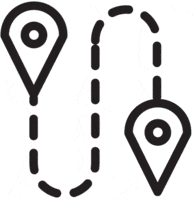# Math Worksheets Land

Math Worksheets For All Ages

# Math Worksheets Land

Math Worksheets For All Ages

Home > Math Topics > Place Value >

# Arrange Place Values Worksheets

This is one of those topics where you may not see a great deal of real-world connection. The focus of this topic is not to be some cool critical thinking or brain teasing activity. The purpose is to ensure that students learn to master all aspects of place value and quickly grasp the impact or level of significance of a digit that is found within a value. This will help students learn to make much quicker and accurate estimates and ultimately lead to better operations-based calculations. These worksheets and lessons show students how to move integers around in a number to make the greatest and least possible value.

### Aligned Standard: 4.NBT.A.2

• Answer Keys - These are for all the unlocked materials above.

### Homework Sheets

Five homework problems should give you a full overview of how your students are doing with this topic.

• Homework 1 - To make the largest value out of any number, move the largest digits into the largest place values.
• Homework 2 - Rearrange number to make the largest value possible.

### Practice Worksheets

I admit that I got carried away with the clip art on these. My stock art company just gave me a bunch of new pics.

• Practice 1 - In this number we will order the digits from least to greatest.
• Practice 2 - Rearrange number to make the smallest value possible.
• Practice 3 - To create the smallest values possible, you want to have the lowest digits at the highest place values.

### Math Skill Quizzes

We keep it a grade 4 skill by limited it to four digits throughout.

• Quiz 1 - Remember the largest value just lists the integers in order big to little (left to right).
• Quiz 2 - The smallest value just reverses that.
• Quiz 3 - See how you make out with this quiz.

### In the Whole Number, How Do You Find the Greatest and Least Place Value?As adults we often forgot how larger values appear to youngsters. A string of 7 to 10 digits can be a bit overwhelming. When they see the values 457829123 and 457892123 can easily look like the same, while the later is a much greater value of the two. The location of where a digit resides is key towards determining its overall value Every digit in the number has a unique value, and place value is a concept that helps us understand it.

We use the base ten numbering system where every successive digit is ten times greater than the digit found to the right of it. This becomes evident when we write a value in expanded form. Expanded form is just a way of writing the explicit value of each digit in a value. Take a look at this by exploring the value: 52,987. When writing in expanded form that becomes:

50,000 + 2,000 + 900 + 80 + 7

For instance, in the number 52,987, the digit (2) represents the second largest value out of all 5 digits that are present even though it is the smallest overall digit.

Now that we understand the significance of these digits how do we manipulate the digits in a number to rearrange them to make the largest and smallest values possible?

If you want to have a larger value by arranging the digits in a value arrange the larger digits at the highest places possible. This means to sequence the digits in descending order (high to low) from left to right. If you want to create the smallest value possible, just do the opposite. T This means to sequence the digits in ascending order (low to high) from left to right. For example, what if we were asked to rearrange the digit in the value (19,275) to the greatest and least values possible.

Greatest: 97,521 (high to low)

Least: 12,579 (low to high)

Quick Trick: If you figure out one, just write the other one as the reverse of it.

Unlock all the answers, worksheets, homework, tests and more!
Save Tons of Time! Make My Life Easier Now

## Thanks and Don't Forget To Tell Your Friends!

I would appreciate everyone letting me know if you find any errors. I'm getting a little older these days and my eyes are going. Please contact me, to let me know. I'll fix it ASAP.